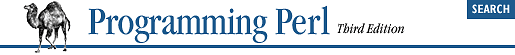home | O'Reilly's CD bookshelfs | FreeBSD | Linux | Cisco | Cisco Exam## 3.13. Bitwise Operators

Like C, Perl has bitwise AND, OR, and XOR (exclusive OR) operators: &, |, and ^. You'll have noticed from your painstaking examination of the table at the start of this chapter that bitwise AND has a higher precedence than the others, but we've cheated and combined them in this discussion.

These operators work differently on numeric values than they do on strings. (This is one of the few places where Perl cares about the difference.) If either operand is a number (or has been used as a number), both operands are converted to integers, and the bitwise operation is performed between the two integers. These integers are guaranteed to be at least 32 bits long, but can be 64 bits on some machines. The point is that there's an arbitrary limit imposed by the machine's architecture.

If both operands are strings (and have not been used as numbers since they were set), the operators do bitwise operations between corresponding bits from the two strings. In this case, there's no arbitrary limit, since strings aren't arbitrarily limited in size. If one string is longer than the other, the shorter string is considered to have a sufficient number of 0 bits on the end to make up the difference.

For example, if you AND together two strings:

`"123.45" & "234.56"`
you get another string:
`"020.44"`
But if you AND together a string and a number:
`"123.45" & 234.56`
The string is first converted to a number, giving:
`123.45 & 234.56`
The numbers are then converted to integers:
`123 & 234`
which evaluates to 106. Note that all bit strings are true (unless they result in the string "0"). This means if you want to see whether any byte came out to nonzero, instead of writing this:
`if ( "fred" & "\1\2\3\4" ) { ... }`
you need to write this:
`if ( ("fred" & "\1\2\3\4") =~ /[^\0]/ ) { ... }`